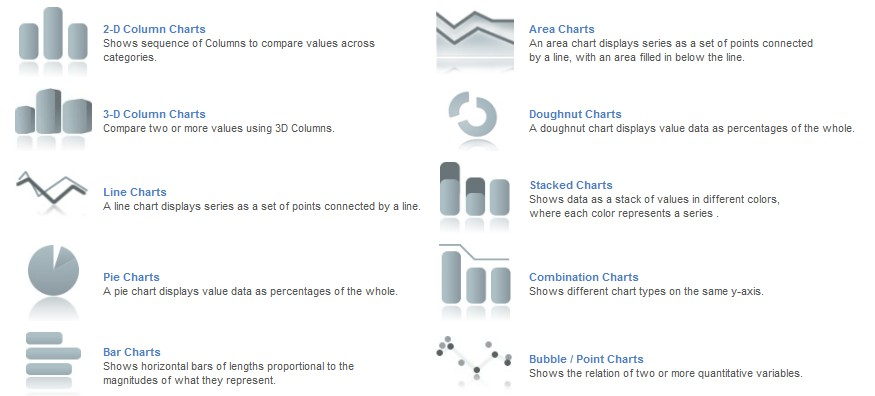## visifire使用心得

visifire是silverlight下很不错的一套开源组件,最近其 发布了2.0beta版本,本文将主要记录一些这个版本跟以前版本不 同的地方.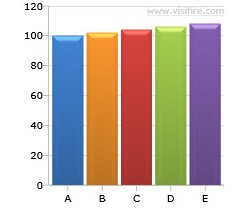visifire为我们提供了一个属性,StartFromZero,通过设置这个属性为false,就可以帮助我们观察到数据的差异,效果如下图: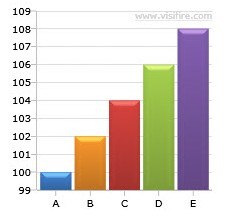Codeprivate
void CreateChart()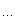{// Create a Chart elementChart chart =
new Chart();// Set chart width and heightchart.Width =
400;chart.Height
=
300;// Create new DataSeriesDataSeries dataSeries =
new DataSeries();// Number of DataPoints to be generatedint numberOfDataPoints =
10;// To set the YValues of DataPointRandom random =
new Random();// Loop and add a few DataPointsfor (int loopIndex =
0; loopIndex < numberOfDataPoints; loopIndex++){// Create a DataPointDataPoint dataPoint =
new DataPoint();// Set the YValue using random numberdataPoint.YValue = random.Next(1, 100);// Add DataPoint to DataSeries}// Add DataSeries to Chart// Add chart to the LayoutRoot for display}Code
for (Int32 i =
0; i <
5; i++)
{

// Update DataPoint YValue property
chart.Series.DataPoints.YValue = rand.Next(-80, 100); // Changing the dataPoint YValue at runtime
}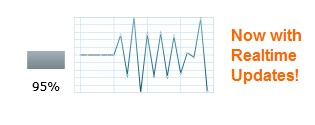Code
dataSeries.RenderAs = RenderAs.Line;

Member name
Area
Bar
Bubble
Column
Doughnut
Line
Pie
Point
StackedArea
StackedArea100
StackedBar
StackedBar100
StackedColumn

StackedColumn100